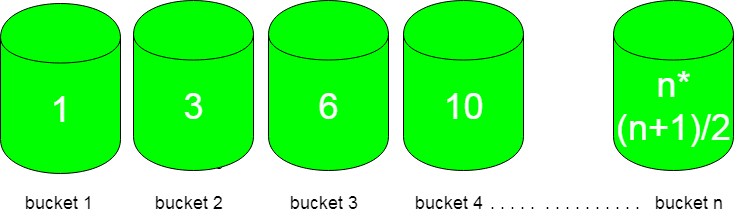GFG App
Open AppBrowser
Continue

# Smallest triangular number larger than p

Given n number of buckets and each bucket is numbered from 1 to n and flowers in it are equal to triangular numbers. You have to choose the bucket which is left with minimum flower after picking ‘p’ flowers from it.First bucket contains only 1 flower, second bucket contains 3, third bucket contains 6 and so on following a pattern of n(n+1)/2.
Examples :

```Input : p = 4
Output : bucket 3
Explanation :
Buckets with flowers : 1 3 6 10 ....
So, bucket 3 is left with only two flowers after
selecting p flowers from it which is minimum.

Input : p = 10
Output : bucket 4
Explanation :
Bucket with flowers : 1 3 6 10 15 ...
So, selecting 10 flowers from 4th bucket leave
it with 0 flowers.```

Approach :
Observing the input/output of different cases, bucket number can be calculated using formula :

`n = ceil( (sqrt(8*p+1)-1)/2 ) ;`

How does it work?
We need smallest n such than n*(n+1)/2 >= p
So we need to find roots of equation n2 + n – 2*p >= 0.
By applying the formula discussed here, we get n = ceil( (sqrt(8*p+1)-1)/2 )

## C++

 `// CPP code to find the bucket to choose ` `// for picking flowers out of it` `#include` `using` `namespace` `std;`   `int` `findBucketNo(``int` `p)` `{` `   ``return` `ceil``( ( ``sqrt``( 8*p + 1 ) -1 ) / 2 ) ;` `}`   `// Driver code` `int` `main()` `{` `  ``int` `p = 10 ;    ` `  ``cout << findBucketNo(p);` `  ``return` `0;` `} `

## Java

 `//Java code to find the bucket to choose ` `// for picking flowers out of it` `import` `java.lang.System.*;`   `class` `GFG {` `    `  `    ``static` `int` `findBucketNo(``int` `p)` `    ``{` `        ``return` `(``int``)Math.ceil(( ` `        ``Math.sqrt( ``8``*p + ``1` `) -``1` `) / ``2` `) ;` `    ``}` `    `  `    ``// Driver code` `    ``public` `static` `void` `main(String[] args)` `    ``{` `        ``int` `p = ``10` `; ` `        `  `        ``System.out.println(findBucketNo(p));` `    ``}` `}`   `// This code is contributed by` `// Smitha Dinesh Semwal`

## Python 3

 `# Python 3 code to find the bucket ` `# to choose for picking flowers` `# out of it` `import` `math`   `def` `findBucketNo(p):`   `    ``return` `math.ceil( ( math.sqrt(` `               ``8``*``p ``+` `1` `) ``-``1` `) ``/` `2` `) `   `# Driver code` `p ``=` `10`   `print``(findBucketNo(p))`   `# This code is contributed by` `# Smitha Dinesh Semwal`

## C#

 `// C# code to find the bucket to choose ` `// for picking flowers out of it` `using` `System;`   `class` `GFG {` `    `  `    ``static` `int` `findBucketNo(``int` `p)` `    ``{` `        ``return` `(``int``)Math.Ceiling(( ` `        ``Math.Sqrt( 8*p + 1 ) -1 ) / 2 );` `    ``}` `    `  `    ``// Driver code` `    ``static` `public` `void` `Main ()` `    ``{` `        ``int` `p = 10 ; ` `        ``Console.WriteLine(findBucketNo(p));` `    ``}` `}`   `// This code is contributed by Ajit.`

## PHP

 ``

## Javascript

 ``

Output :

`4`

Time Complexity : O(logn)

Auxiliary Space: O(1) as it is using constant space for variables

My Personal Notes arrow_drop_up